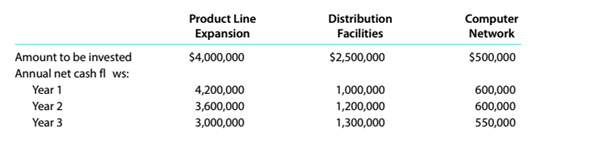Chapter 15, Problem 15.3.1P

Chapter
Section
Textbook Problem

Net present value method, present value index, and analysisDonahue Industries Inc. wishes to evaluate three capital investment projects by using thenet present value method. Relevant data related to the projects are summarized as follows:InstructionsAssuming that the desired rate of Si urn is 20%, preparea net present value analysts for eachproject. Use the present value of Si table appearing in this chapter.

To determine

Concept Introduction:

NPV:

Net present value (NPV) is the method to evaluate the project feasibility. This method calculates the present value of cash inflows and outflows, and then calculates the net present value of the investment. A project should be accepted if it has a positive NPV. The formula to calculate the NPV is as follows:

NPV = Present value of cash inflows  Present value of cash out flows

To Prepare:

The Net Present value analysis for each project

Explanation

The Net Present value analysis for each project is prepared as follows:

 Product Line Expansion Amount PVF (20%) PV A B =A*B Annual Net Cash Flows Year 1 $4,200,000 0.83333$ 3,500,000 Year 2 $3,600,000 0.69444$ 2,500,000 Year 3 $3,000,000 0.57870$ 1,736,111 Present Value of Cash Inflow (A) $7,736,111 Investment (B)$ 4,000,000 1.00000 $4,000,000 Net Present Value (A-B)$ 3,736,111 Distribution Facilities Amount PVF (20%) PV A B =A*B Annual Net Cash Flows Year 1 $1,000,000 0.83333$ 833,333 Year 2 \$ 1,200,000 0...

Still sussing out bartleby?

Check out a sample textbook solution.

See a sample solution

The Solution to Your Study Problems

Bartleby provides explanations to thousands of textbook problems written by our experts, many with advanced degrees!

Get Started

Find more solutions based on key concepts